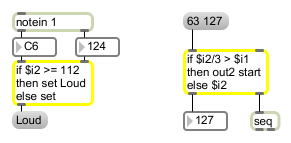# if

Conditional statement in if/then/else form

## Description

if evaluates input according to a conditional statement specified in an if-then-else form.

## Arguments

Name Type Opt Description
if symbol Obligatory. The arguments for the if object start with a conditional statement that uses the same syntax as expr. Refer to the description of the expr object for details. The word then follows the conditional statement, which is then followed by a message expression. After the message expression, there is an optional else and a second message expression.

if evaluates the conditional expression, and if the result is non-zero, evaluates the message expression after the word then. Otherwise, it evaluates the second message expression after the word else (or does nothing in the case where no else and second message expression have been typed in.
then else symbol Message expressions are similar to what you type into a message box, with the following differences:
\$i1 \$f1 \$s1 symbol You use \$i1, \$f1, or \$s1 instead of \$1 for changeable arguments.
send symbol No commas or semicolons are allowed. Messages can be sent to remote receive objects by preceding the message expression with send, followed by the name of the receive object.
out2 symbol The keyword out2 in a message expression creates a second, right outlet for the if object. If out2 precedes a message expression, the result of the expression is sent out the right outlet instead of the left outlet.

## Messages

 bang In left inlet: Evaluates the conditional statement using the values currently stored. Any of the above messages in the left inlet will evaluate the conditional statement and send out the result. Any inlets which have not yet received a value have the value 0 by default. The number of inlets is determined by how many different changeable arguments are typed in. The maximum number of inlets is 9. int input [int] The number in each inlet will be stored in place of the \$i or \$f argument associated with it. (Example: The number in the second inlet from the left will be stored in place of the \$i2 and \$f2 arguments, wherever they appear.) (inlet1) input [int] Performs the same function as int. (inlet2) input [int] Performs the same function as int. (inlet3) input [int] Performs the same function as int. (inlet4) input [int] Performs the same function as int. (inlet5) input [int] Performs the same function as int. (inlet6) input [int] Performs the same function as int. (inlet7) input [int] Performs the same function as int. (inlet8) input [int] Performs the same function as int. (inlet9) input [int] Performs the same function as int. float input [float] The number in each inlet will be stored in place of the \$f or \$i argument associated with it. The number will be truncated by a \$i argument. (inlet1) input [float] Performs the same function as float. (inlet2) input [float] Performs the same function as float. (inlet3) input [float] Performs the same function as float. (inlet4) input [float] Performs the same function as float. (inlet5) input [float] Performs the same function as float. (inlet6) input [float] Performs the same function as float. (inlet7) input [float] Performs the same function as float. (inlet8) input [float] Performs the same function as float. (inlet9) input [float] Performs the same function as float. set set-input [list] In left inlet: The word set, followed by one or more numbers, treats those numbers as if each had come in a different inlet, replacing the stored value with the new value, but the conditional statement is not evaluated and nothing is sent out the outlet. If there are fewer numbers in the message than there are inlets, the stored value in each remaining inlet is left unchanged. symbol input [list] In left inlet: The word symbol, followed by a symbol (a word), will be stored in place of the \$s argument.

## Output

anything: The message after the then or else portion of the arguments is sent out the outlet. If the word out2 is present as an argument, there will be two outlets, and messages following out2 will be sent out the right outlet. If the word send is present as an argument, the word that follows it is the name of a receive object, and the message that follows it will be sent to receive objects with that name.

## ExamplesComplex comparisons and results can be described in a single object